# Linear representation theory of general affine group of degree two over a finite field

## Contents

This article gives specific information, namely, linear representation theory, about a family of groups, namely: general affine group of degree two.
View linear representation theory of group families | View other specific information about general affine group of degree two

## Summary

Item Value
degrees of irreducible representations over a splitting field 1 ($q - 1$ times),$q$ ($q - 1$ times),$q + 1$ ($(q - 1)/(q - 2)/2$ times),$q - 1$ (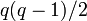$q(q - 1)/2$ times),$(q^2 - 1)$ ($(q - 1)$ times),$(q^2 - 1)(q - 1)$ (1 time)
number of irreducible representations$q^2 + q - 1$, equal to the number of conjugacy classes. See number of irreducible representations equals number of conjugacy classes, element structure of general affine group of degree two over a finite field#Conjugacy class structure
maximum degree of irreducible representation over a splitting field$(q^2 - 1)(q - 1)$
lcm of degrees of irreducible representations over a splitting field Case$q$ odd:$q(q^2 - 1)(q-1)/2$; case$q$ even: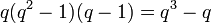$q(q^2 - 1)(q-1) = q^3 - q$
sum of squares of degrees of irreducible representations over a splitting field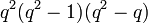$q^2(q^2 - 1)(q^2 - q)$, equal to the order of the group. See sum of squares of degrees of irreducible representations equals group order.

## Irreducible representations

Below are the irreducible representations over the field of complex numbers. These work over any splitting field of characteristic zero or, more generally, of characteristic coprime to the order of the group.

Description of collection of representations Parameter for describing each representation How the representation is described Degree of each representation Number of representations Sum of squares of degrees
Descends to$GL(2,q)$: One-dimensional, factor through the determinant map a homomorphism$\alpha: \mathbb{F}_q^\ast \to \mathbb{C}^\ast$$x \mapsto \alpha(\det x)$ 1$q - 1$$q - 1$
Descends to$GL(2,q)$: Unclear a homomorphism$\varphi:\mathbb{F}_{q^2}^\ast \to \mathbb{C}^\ast$, up to the equivalence$\! \varphi \simeq \varphi^q$, excluding the cases where$\varphi = \varphi^q$ unclear$q - 1$$q(q - 1)/2$$q(q - 1)^3/2 = (q^4 - 3q^3 + 3q^2 - q)/2$
Descends to$GL(2,q)$: Tensor product of one-dimensional representation and the nontrivial component of permutation representation of$GL_2$ on the projective line over$\mathbb{F}_q$ a homomorphism$\alpha: \mathbb{F}_q^\ast \to \mathbb{C}^\ast$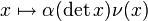$x \mapsto \alpha(\det x)\nu(x)$ where$\nu$ is the nontrivial component of permutation representation of$GL_2$ on the projective line over$\mathbb{F}_q$$q$$q - 1$$q^2(q - 1) = q^3 - q^2$
Descends to$GL(2,q)$: Induced from one-dimensional representation of Borel subgroup$\alpha, \beta$ homomorphisms$\mathbb{F}_q^\ast \to \mathbb{C}^\ast$ with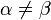$\alpha \ne \beta$, where$\{ \alpha, \beta \}$ is treated as unordered. Induced from the following representation of the Borel subgroup:$\begin{pmatrix} a & b \\ 0 & d \\\end{pmatrix} \mapsto \alpha(a)\beta(d)$$q + 1$$(q - 1)(q - 2)/2$$(q + 1)^2(q - 1)(q - 2)/2 = (q^4 - q^3 - 3q^2 + q + 2)/2$
?  ?  ?$q^2 - 1$$q - 1$$(q^2 - 1)^2(q - 1)$
?  ?  ?$(q^2 - 1)(q - 1)$ 1$(q^2 - 1)^2(q - 1)^2$
Total NA NA NA$q^2 + q - 1$$q^2(q^2 - 1)(q^2 - q)$